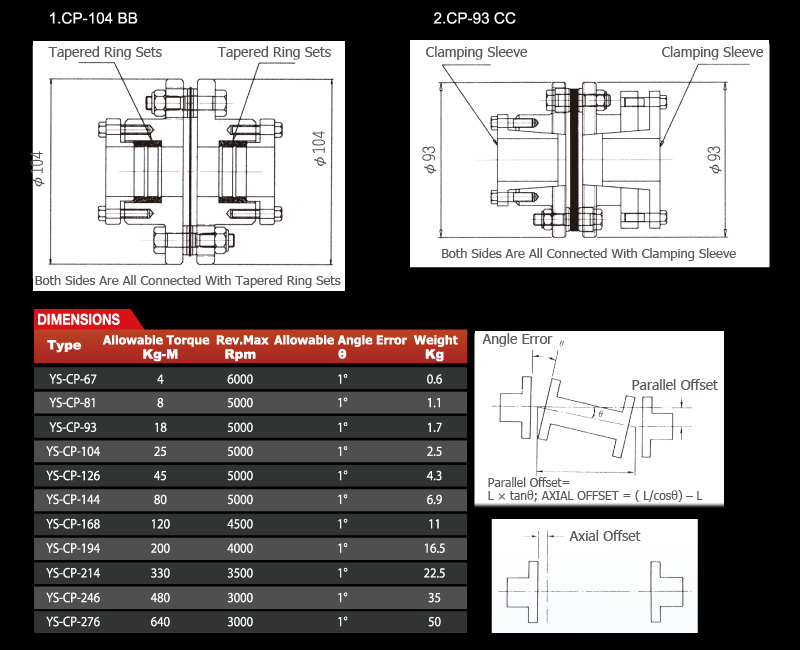Flex Coupling
•• To Select The Right Type Of Flex Coupling
1. Select the load factor lf from table 1 basis on the driven machine you want to use.
2. Calculate the torque ta from formula ta (kgf-m) = 974*p(kw)/n(rpm)*lf where p is input power, n is input rotary speed, lf is load factor.
3. Select the right type of flex coupling from specifications which allowable torque Tw is bigger than Ta. also check the Dmax from dimensions if it is bigger than the shaht bore diameter you want to use.
Example for Selection
Example：
Input power p is 120 kw, driven machine is centrifugal pump,input rotary speed n is 1800 prm, shaft bore diameter d is 50 mm,connected with key on both sides, try to select the right type of flex coupling.
Sol：
1. Select the load factor lf = 1.5 from table 1.
2. Calculate ta = 974*p/n*lf = 974*120/1800*1.5 = 64.9 kgf-m
3. Select the right type of flex coupling. that is cp-144 which allowable torque tw = 80 kgf-m is bigger than ta = 64.9kgf. also dmax = 60 mm of cp-144 is bigger than the shaft bore diameter d=50mm.